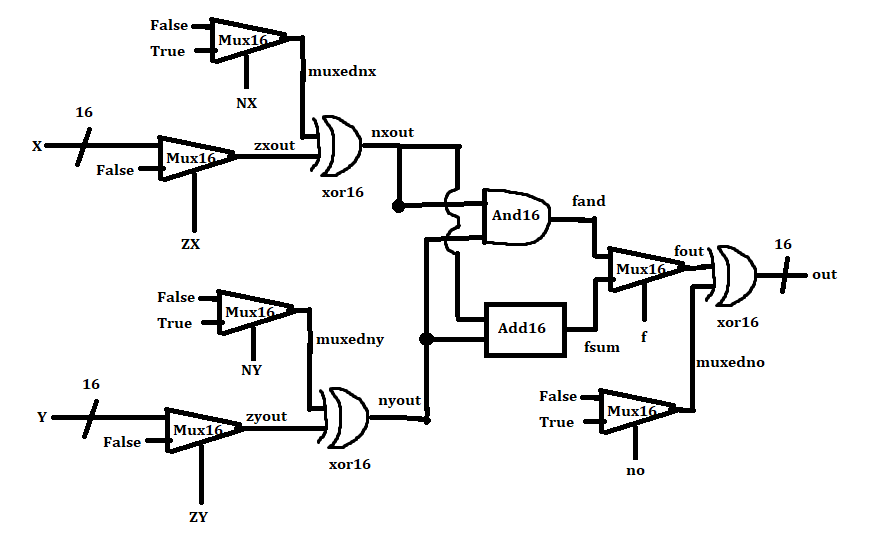# Someone can see what I did wrong?ClassicListThreaded2 messagesHello, thank you very much for reading my question. I have been trying for the last week to build an ALU, but I keep getting the comparison error. I attached an image of my design (sorry for my bad drawing). As you can see, I built a "xor16" for this project. Can someone see where the error is? I would be very grateful if someone finds it. Thank you so much.PARTS:     Mux16(a=x[0..15], b=false, sel=zx, out=zxout);     Mux16(a=false, b=true, sel=nx, out=muxednx);         Xor16(a=muxednx, b=zxout, out=nxout);     Mux16(a=y[0..15], b=false, sel=zy, out=zyout);         Mux16(a=false, b=true, sel=ny, out=muxedny);         Xor16(a=muxedny, b=zyout, out=nyout);     And16(a=nxout, b=nyout, out=fand);     Add16(a=nxout, b=nyout, out=fsum);     Mux16(a=fand, b=fsum, sel=f, out=fout);     Mux16(a=false, b=true, sel=no, out=muxedno);     Xor16(a=fout, b=muxedno, out=out[0..15]);